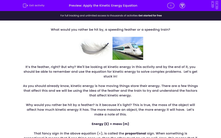# Apply the Kinetic Energy Equation

In this worksheet, students will develop their knowledge of how to use the kinetic energy equation in more complex problems.Key stage:  KS 4

Year:  GCSE

GCSE Boards:   AQA, AQA Trilogy, AQA Synergy, OCR 21st Century, OCR Gateway, Pearson Edexcel, Eduqas,

Curriculum topic:   Energy, Movement and Interactions, Explaining Motion, Conservation of Energy

Difficulty level:#### Worksheet Overview

What would you rather be hit by, a speeding feather or a speeding train?It’s the feather, right? But why? We'll be looking at kinetic energy in this activity and by the end of it, you should be able to remember and use the equation for kinetic energy to solve complex problems.  Let’s get stuck in!

As you should already know, kinetic energy is how moving things store their energy. There are a few things that affect this and we will be using the idea of the feather and the train to try and understand the factors that affect kinetic energy.

Why would you rather be hit by a feather? Is it because it's light? This is true, the mass of the object will affect how much kinetic energy it has. The more massive an object, the more energy it will have.  Let’s make a note of this.

Energy (E) ∝ mass (m)

That fancy sign in the above equation (∝), is called the proportional sign. When something is proportional it means that if one thing goes up, then the other must go up as well. Here, this means that if we change the mass, we also change the energy.

Let’s change the situation a little bit now. The train is moving at 0.01 m/s and the feather is moving at 1,000 m/s. Which one would you rather be hit by now? Did you say the train? That’s because speed (or velocity) also has an effect on the kinetic energy. Let’s add this to our proportionality:

Energy (E) ∝ mass (m) x velocity (v)

All we are saying here is that if we change either the mass or the velocity, then it will affect how much energy there is. If either of them goes up, then the energy will also go up and vice-versa, if they go down.

Unfortunately, it isn’t as simple as all that… scientists have conducted experiments and found that one of these things has a much more significant effect on energy than the other. When we draw graphs of energy against mass and velocity, we find that the mass graph forms a line of about y = ½ x and the velocity graph looks like a y = x2 graph!

This leads us to the equation - we have to halve the mass and square the velocity so the equation looks like this:Fun fact time! You have to remember this equation - you will not be given in the exam!

So how do you use this equation? Simple! Let’s go through an example together:

Jim has a kit bag that has a mass of 5 kg and has just done a sprint at the gym burning 18 calories. He is now walking home from the gym at a speed of 2.3 m/s with his kit bag. What is his kinetic energy? Jim has a mass of 80 kg.

Step 1  Find the values that matter to you in this question and highlight them:

Jim has a kit bag that has a mass of 5 kg and has just done a sprint at the gym burning 18 calories. He is now walking home from the gym at a speed of 2.3 m/s with his kit bag. What is his kinetic energy? Jim has a mass of 80 kg.

Step 2  Write out the values next to their symbols. Remember if there is more than one number, then you might have to add them together. Think of the situation in real life and apply that to the equation:

mass = 80 kg + 5 kg = 85 kg

velocity = 2.3 m/s

Step 3   Halve the mass and square the speed:

m = 42.5 kg

v = 5.29 m/s

Step 4   Put these into the equation:

E = 42.5 x 5.29

Step 5   Put these into your calculator and press =

E = 224.825 J

Step 6   Round the answer to one decimal place or 2 significant figures unless the question specifies otherwise!

E = 224.8 J

Don’t forget the unit of energy is the joule (J)!

Now let's have a go at some questions.

### What is EdPlace?

We're your National Curriculum aligned online education content provider helping each child succeed in English, maths and science from year 1 to GCSE. With an EdPlace account you’ll be able to track and measure progress, helping each child achieve their best. We build confidence and attainment by personalising each child’s learning at a level that suits them.

Get started